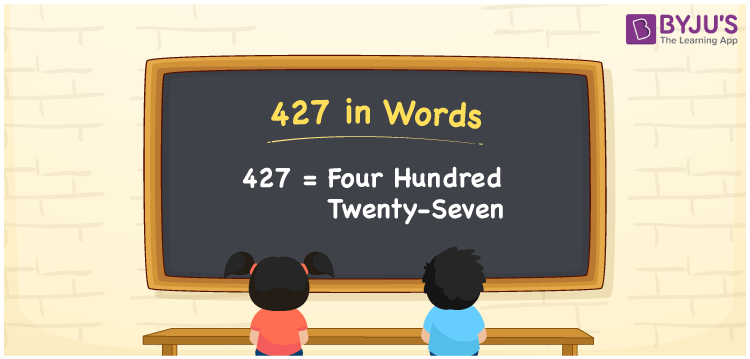# 427 in Words

427 in words is written as Four hundred twenty-seven. In both the International System of Numerals and the Indian System of Numerals, 427 is written as Four hundred twenty-seven. The number 427 is a Cardinal Number as it represents some quantity. For example, the “cost of the t-shirt is 427 rupees”.

 427 in Words Four hundred twenty-seven Four hundred twenty-seven in Number 427

## 427 in English Words

We write 427 in English Words using the letters of the English alphabet. Therefore, we read 427 in English as “Four hundred twenty-seven”.## How to Write 427 in Words?

To write 427 in words, we shall use the place value chart. In the place value chart, write 4 in the hundreds, 2 in the tens, and 7 in the ones, respectively. Now let us make a place value chart to write the number 427 in words.

 Hundreds Tens Ones 4 2 7

Thus, we can write the expanded form as

4 × Hundred + 2 × Ten + 7 × One

= 4 × 100 + 2 × 10 + 7 × 1

= 400 + 20 + 7

= 427

= Four hundred twenty-seven.

427 is a natural number, the successor of 426 and the predecessor of 428.

427 in words – Four hundred twenty-seven

• Is 427 an odd number? – Yes
• Is 427 an even number? – No
• Is 427 a perfect square number? – No
• Is 427 a perfect cube number? – No
• Is 427 a prime number? – No
• Is 427 a composite number? – Yes

## Frequently Asked Questions on 427 in Words

Q1

### How to write 427 in words?

427 in words is written as Four hundred twenty-seven.
Q2

### How to write 427 in the International and Indian System of Numerals?

In both, the system of numerals, 427 in words, is written as Four hundred twenty-seven.
Q3

### What is the successor of 427?

The successor of 427 is 428.